## A simple example

Let’s load the igraph package, create a random graph using the Erdos-Rényi model and derive the corresponding Minimal Spanning Tree (MST).

``````library(igraph)
g <- erdos.renyi.game(10, 3/10)
mst <- minimum.spanning.tree(g)``````

See also `igraph`’s MST documentation

Next we’d like to visualise the graph `g` and the `mst`

``````par(mfrow=c(1,2), mar=c(0,1,0.75,0)) # sub-plots and margins
plot(g , main="Graph")
plot(mst, main = "MST")``````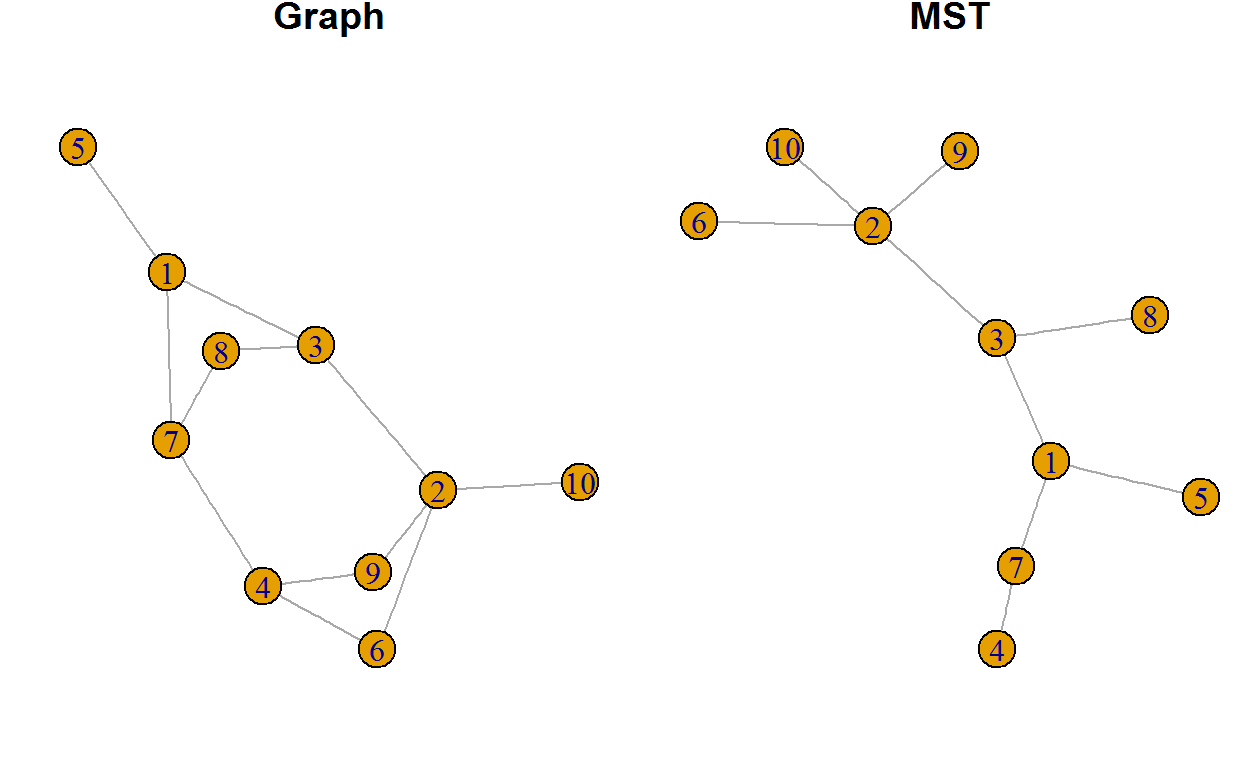## Network

### Definition

Let us assume you want to create your own graph. You have got the following edges:

``````edges <- read.table(textConnection(
"from to weight
1   2   35
1   2   35
2   3   25
2   4   10
3   4   20
3   5   15
4   5   30

Alternatively you might want to specify them as a matrix:

``A = matrix(data=c(1, 1, 2, 2, 3, 3, 4, 2, 3, 3, 4, 4, 5, 5,35,40,25,10,20,15,30),ncol = 3)``

### Visualise

Now let us create the graph and display it.

``````G = graph.data.frame(A[,1:2] ,directed = FALSE) #or use edges
plot(G, asp=0) # asp ... aspect ratio (0=no ratio)``````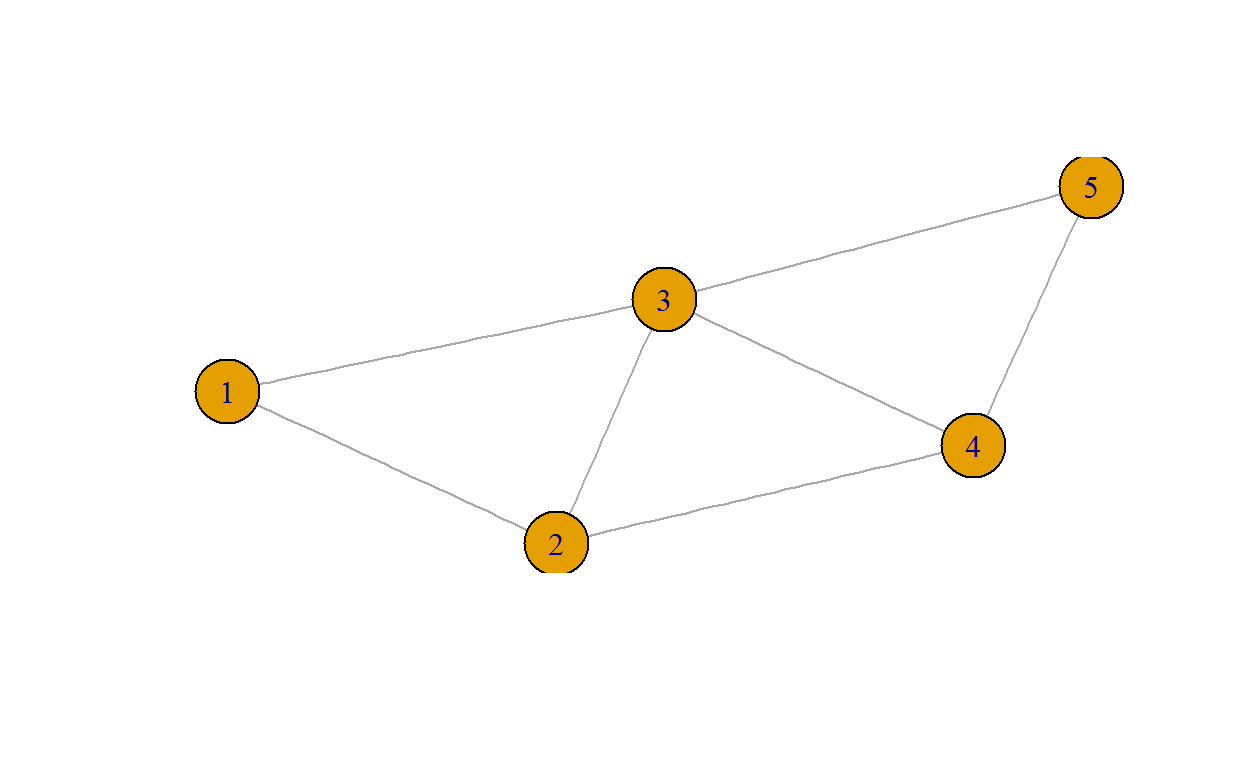Since it is a network the weights have to be displayed. Larger nodes are also nice to have.

``````E(G)\$weight = A[,3]       # specify the actual weight
E(G)\$label  = E(G)\$weight # labels for the weight
V(G)\$size = 40            # node size
plot(G, asp=0)``````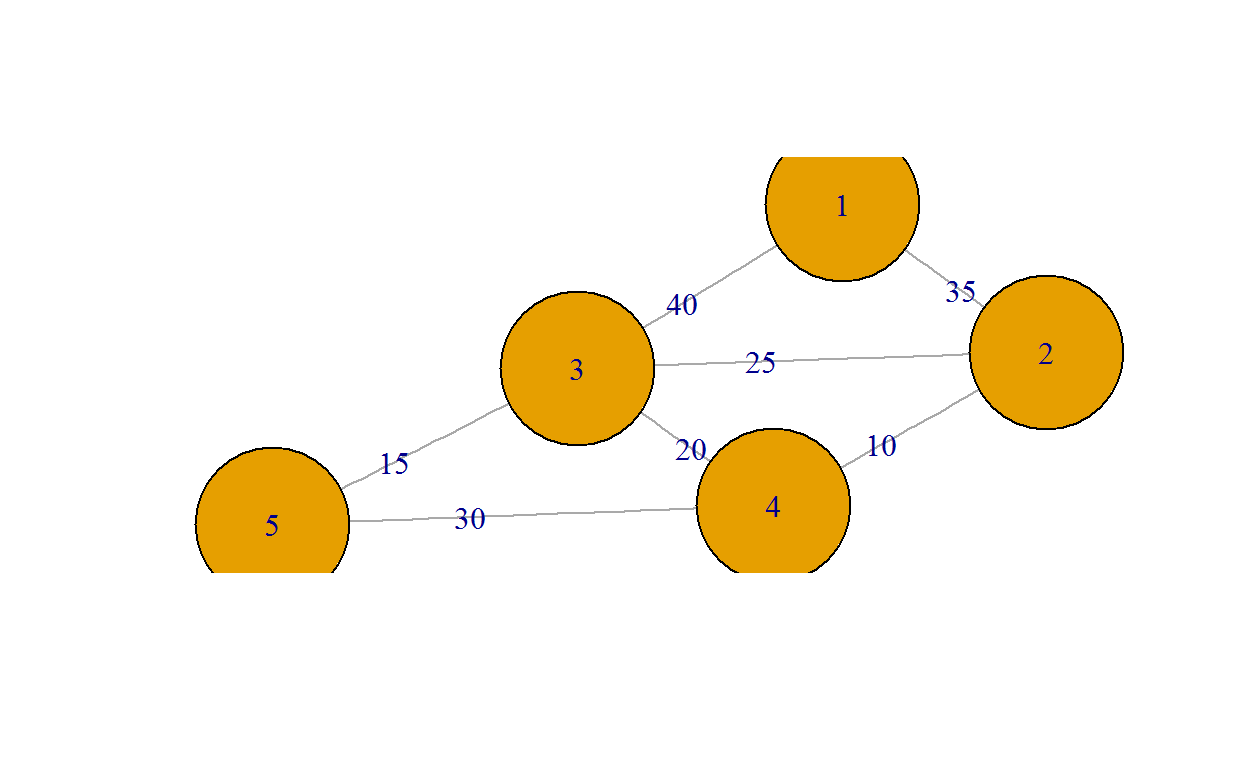It is possible to provide the node and edge label via the plot function.

``````plot(G,
edge.label=c('e1','  e2','  e3','  e4','  e5','  e6','  e7'),
edge.curved=T, edge.width=3, edge.color='black',
edge.label.font=1, edge.label.cex=0.85,
vertex.label=c('one','two','three','four','five'),
vertex.shape='crectangle',vertex.color='gray',
vertex.size=64,vertex.size2=40)``````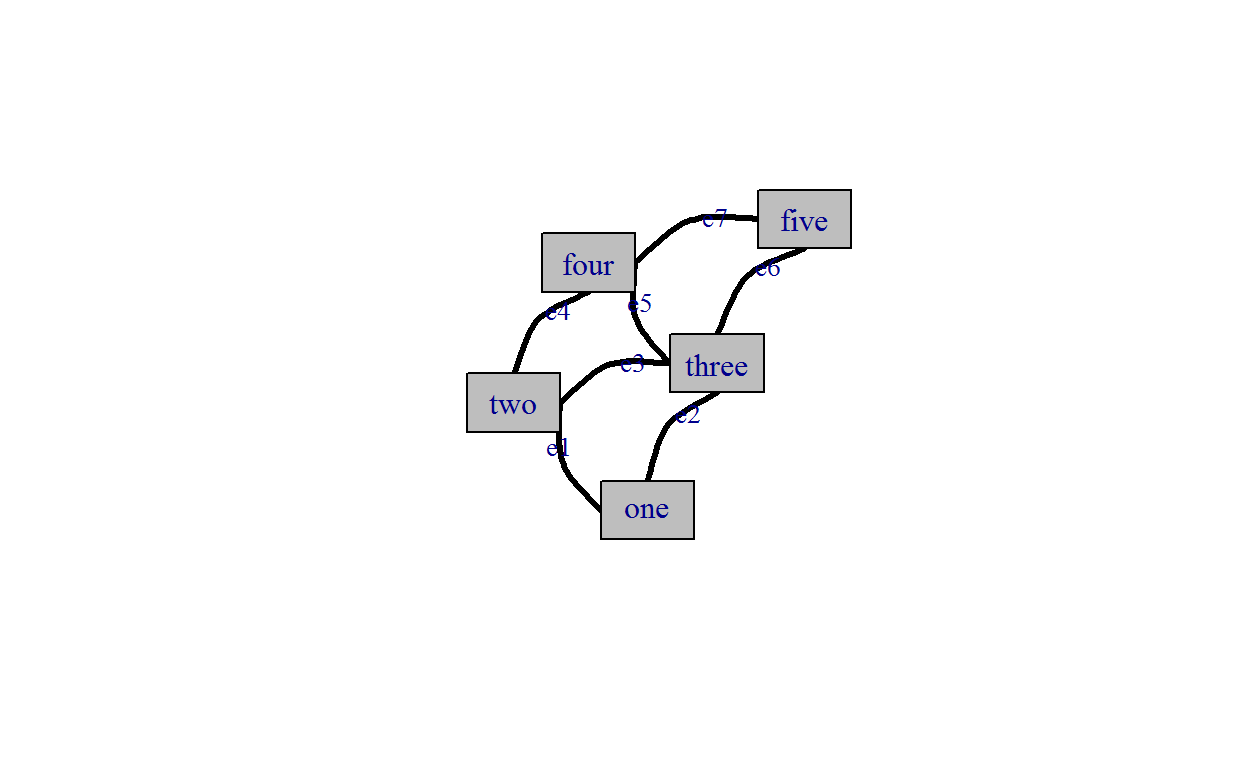The `igraph` contains some typical customisations. By the way the placement of the edge labels might be better done with the text function.

Often it is important to place vertices at predetermined locations. You can use the interactive tool `tkplot` and drag your nodes to desired positions.

``handle = tkplot(G)``

Then you can extract the coordinates with:

``my_layout = tk_coords(handle)``

Now you can provide the layout to the `plot` function.

``plot(G,layout=my_layout)``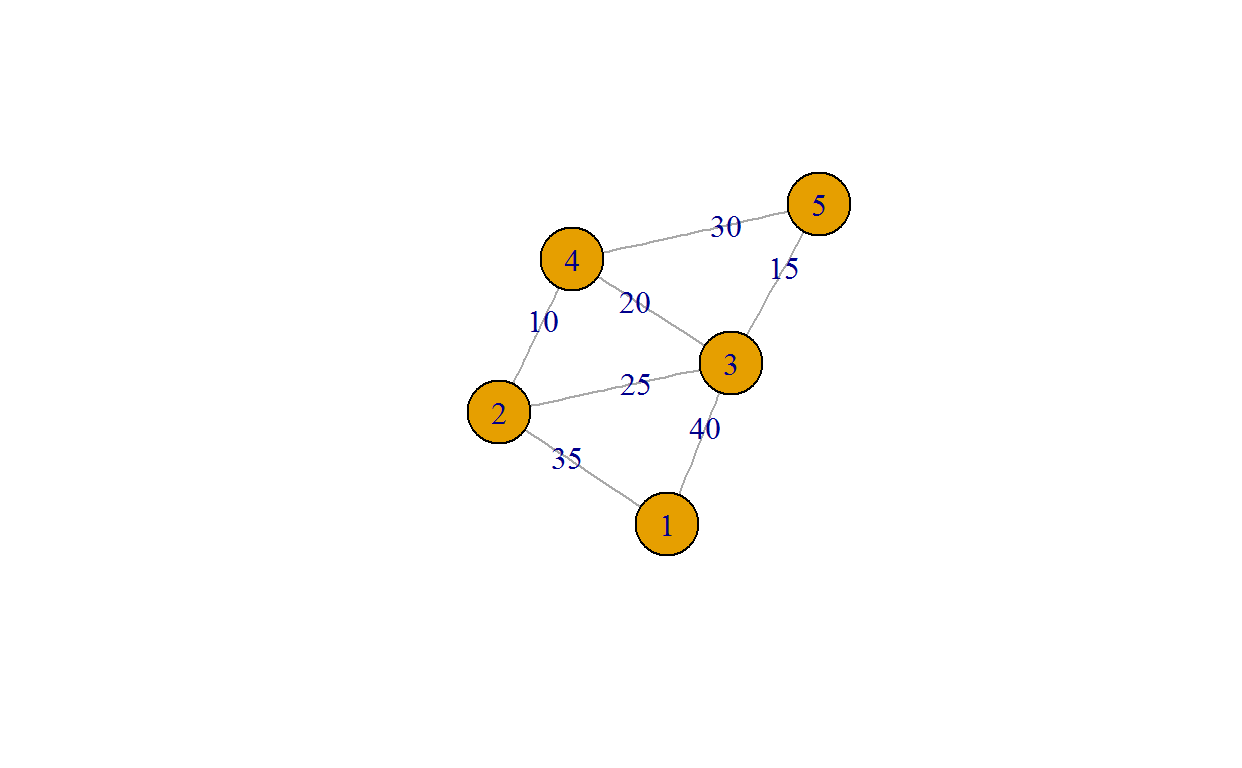In order to place the nodes at predetermined locations specify coordinates:

``````x = c(30,180,180,360,360)
y = c(120,240,0,240,0)
coords = cbind(x,y)
plot(G, layout = coords)``````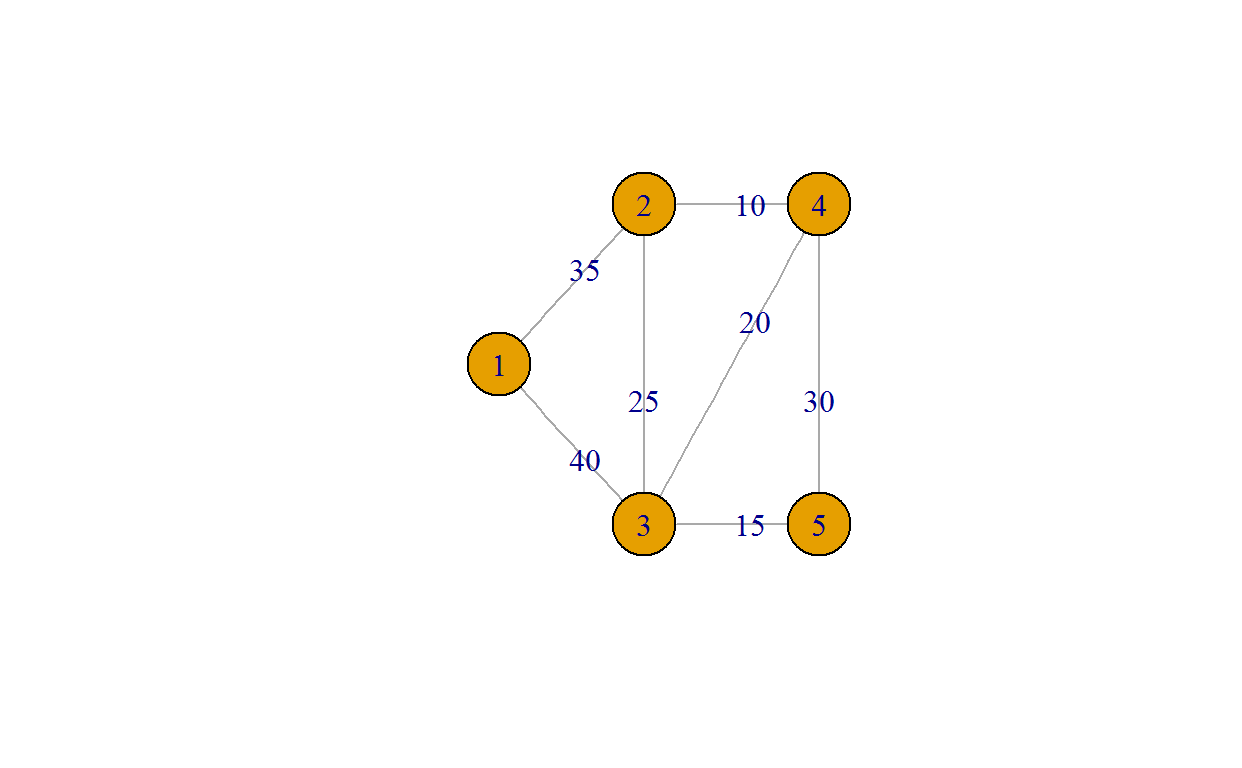Now let us briefly introduce, how to obtain fundamental properties of a graph.

``cat("#nodes =", vcount(G), "; #edges =", ecount(G)) # number of vertices and edges``
``## #nodes = 5 ; #edges = 7``
``V(G) # the individual vertices (nodes)``
``````## + 5/5 vertices, named:
##  1 2 3 4 5``````
``E(G) # all edges``
``````## + 7/7 edges (vertex names):
##  1--2 1--3 2--3 2--4 3--4 3--5 4--5``````
``E(G)\$weight # weights related to all edges``
``##  35 40 25 10 20 15 30``

## Minimal Spanning Tree

Here, we’ll determine the MST and visualise it.

``````mst = minimum.spanning.tree(G)
plot(mst, layout = coords)``````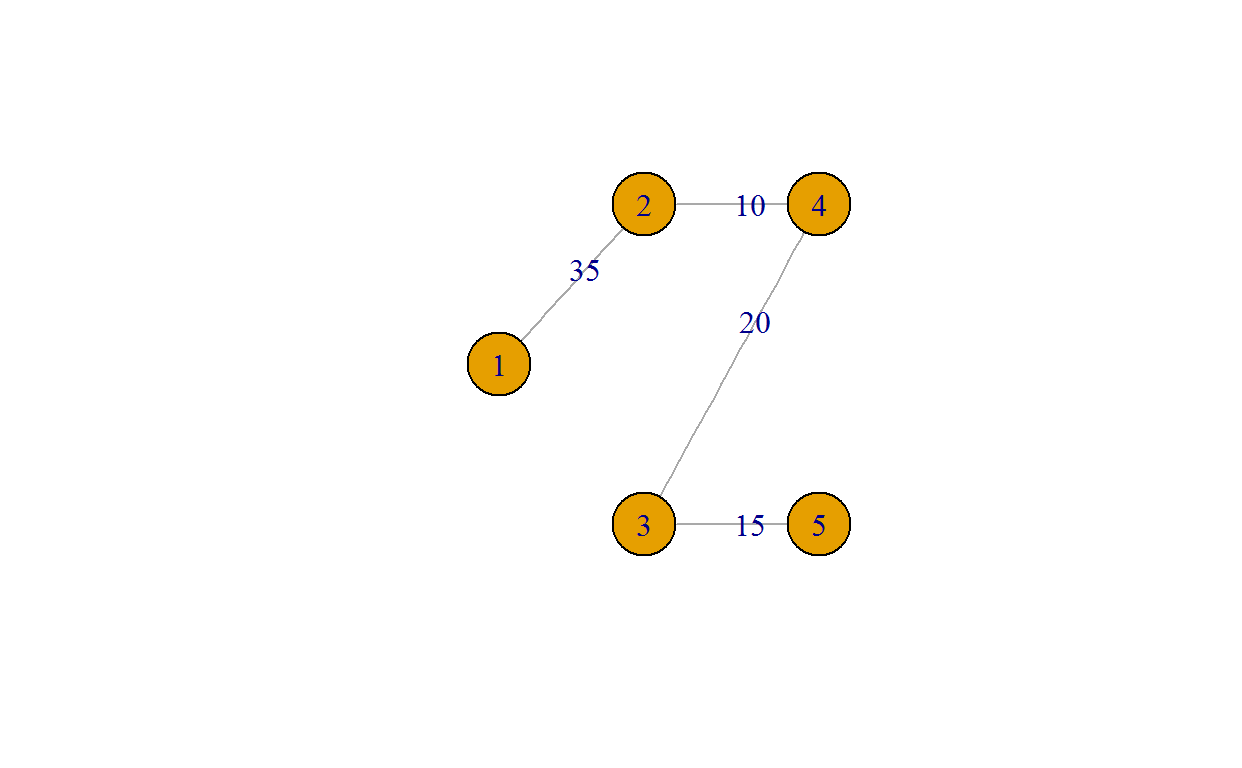Next we will display the MST within the original graph G. In order to do this in a simple way, I will introduce a “helper” function.

``````# Tests whether row vectors are within a matrix; identifies rows in matrix accordingly
vectorsInVectorSeq <- function(testVectorMatrix, seqVectorMatrix) {
r <- rep(FALSE,nrow(seqVectorMatrix))
for (k in 1:nrow(testVectorMatrix)) {
testElement = testVectorMatrix[k,]
r <- r | apply(seqVectorMatrix, 1, function(x, test) isTRUE(all.equal(x, test)), testElement)
}
return(r)
}``````

We apply the above function in the following way

``````e.G    = get.edges(G,1:ecount(G))     # matrix of edges of G
e.mst  = get.edges(mst,1:ecount(mst)) # matrix of edges of MST
mst_idx = vectorsInVectorSeq(e.mst, e.G) # row indicis in G of MST ``````

Now we can finally highlight the MST within the original graph G.

``````ecol <- rep("gray80", ecount(G)) # default edge colour
ecol[mst_idx] <- "gold" # colour for MST

ew <- rep(2,ecount(G)) # default edge width
ew[mst_idx] <- 5 # width for MST edges

plot(G, layout = coords, edge.color=ecol, edge.width=ew)``````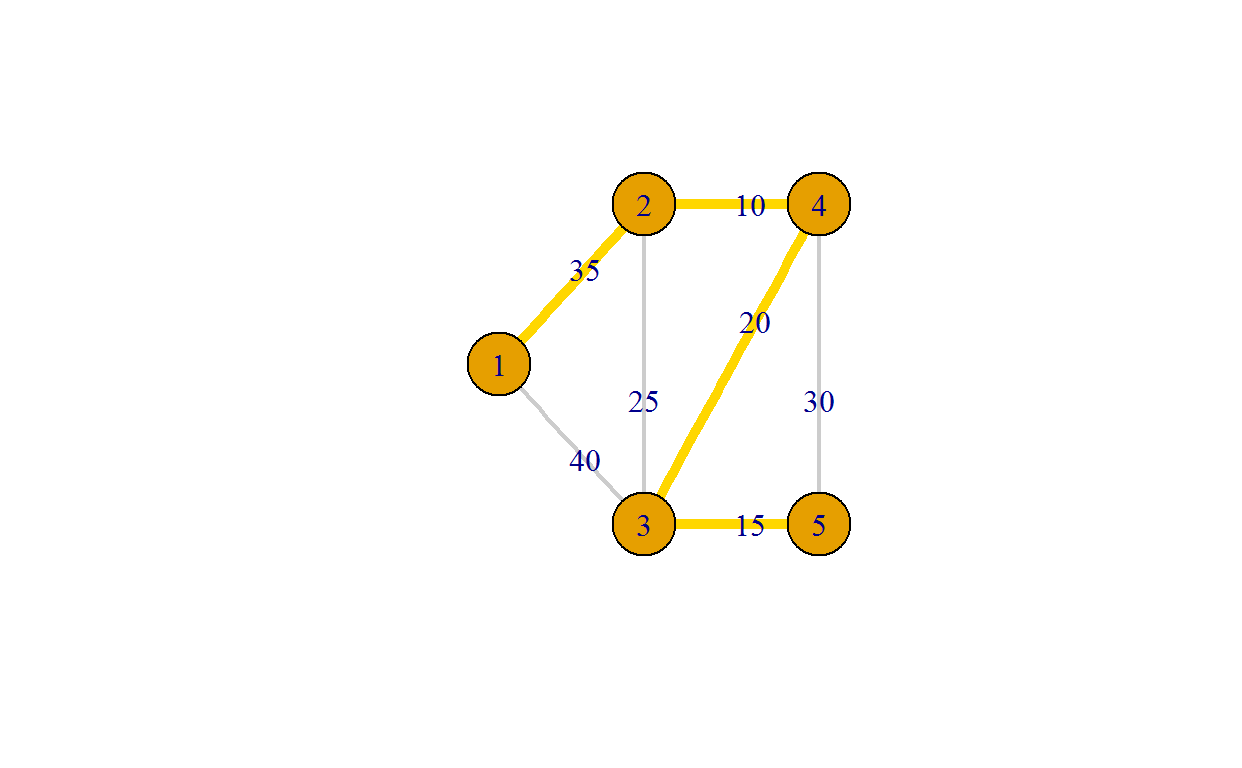## GIS displays

In this section we will show how to map a MST on Google Maps.

``````library(ggmap) # load Google Map package